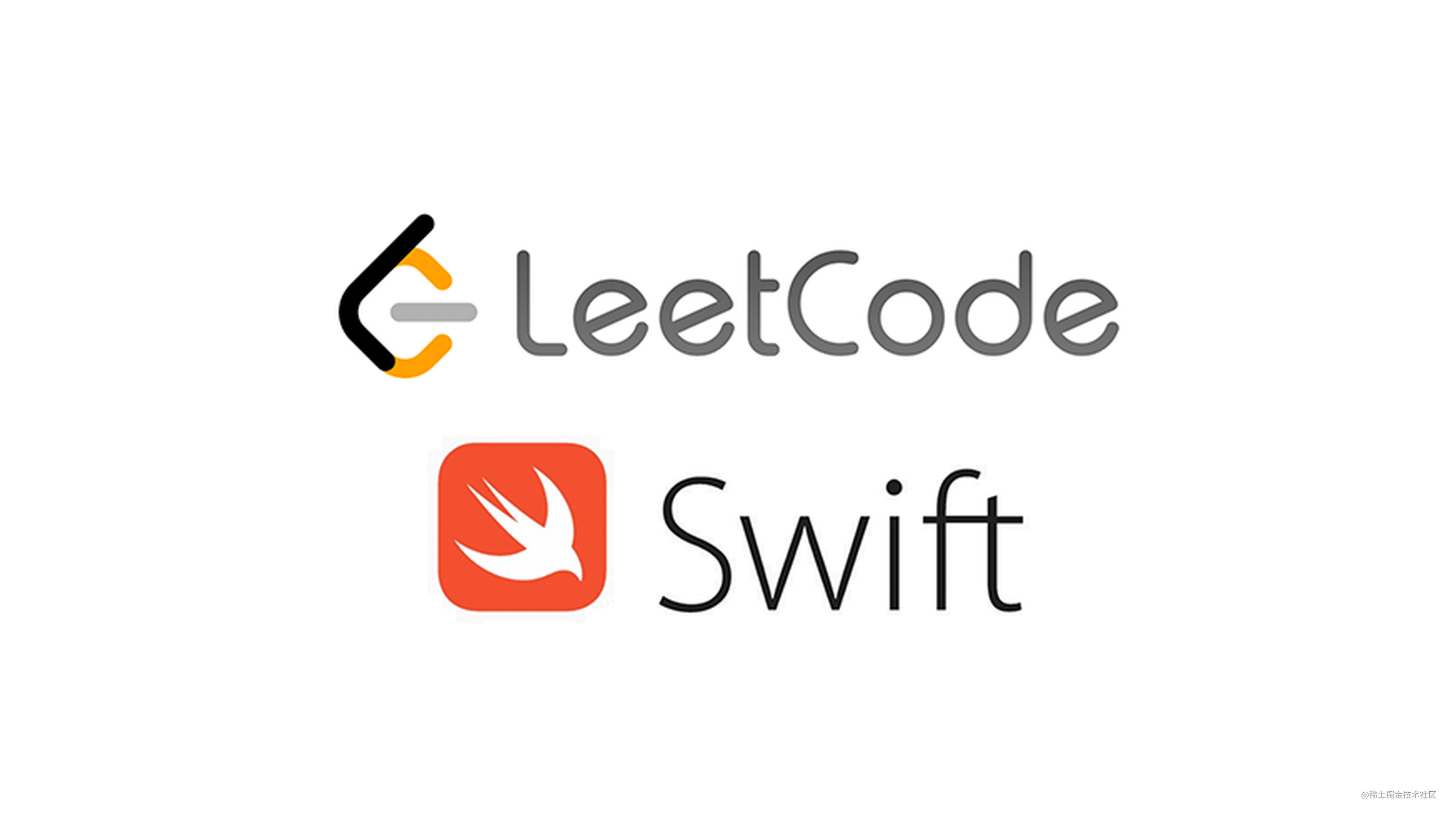# LeetCode - #70 爬楼梯（Top 100）## 前言

LeetCode 算法到目前我们已经更新了 69 期，我们会保持更新时间和进度（周一、周三、周五早上 9:00 发布），每期的内容不多，我们希望大家可以在上班路上阅读，长久积累会有很大提升。

## 2. 示例

``````输入：n = 2

1. 1 阶 + 1 阶
2. 2 阶

``````输入：n = 3

1. 1 阶 + 1 阶 + 1 阶
2. 1 阶 + 2 阶
3. 2 阶 + 1 阶

• `1 <= n <= 45`

## 3. 答案

``````class ClimbingStairs {
func climbStairs(_ n: Int) -> Int {
if n < 0 {
return 0
}
if n == 0 || n == 1 {
return 1
}

var prev = 0, post = 1, total = 0

for i in 1...n {
total = prev + post

prev = post
post = total
}

}
}

• 主要思想：动态编程，`dp = dp + dp[i - 2]`
• 时间复杂度： O(n)
• 空间复杂度： O(1)

iOS

iOS